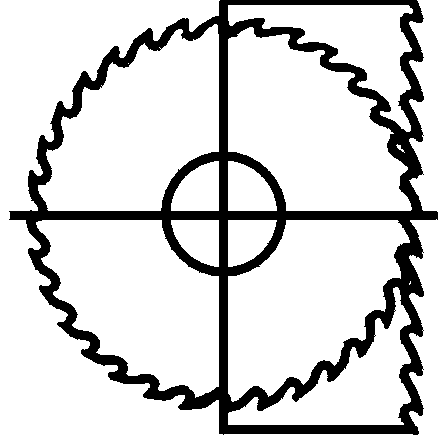thinkerf.com

# Saw Design Formulas

Home     Filer's Forum     Filer's Calculators    Technical Literature     Sawmilling Blog     Contact TKT# Formula sheet

These are the formulas used to design and select circular saws and bandsaws.   For now, only the formulas and the definition of the variables are given.   Later we will add a more complete explanation of what these formulas mean.

### Saw and Tooth Shape

d          Circular saw diameter or bandmill wheel diameter (inch)

z           Distance between centers of bandsaw  wheels

P          Tooth pitch (inch)

n           Number of teeth

k           Kerf

h           Thickness of saw plate

s           Side Clearance (inch)

sMIN     Minimum recommended side clearance (inch)

a           Gullet area (sq. inch)

### Operating Conditions

N          Shaft speed (rpm)

c           Blade ( or rim) speed (sfpm)

b          Bite per tooth (inch)

D         Depth of cut (inch)

f          Feed speed (fpm)

fMAX    Maximum recommended feed speed (fpm)

fMIN     Minimum recommended feed speed (fpm)

### Performance Prediction

GFI          Gullet Feed Index  = Volume of wood removed by a tooth

---------------------------------------------

Volume of tooth gullet

GFIMAX    Maximum allowable Gullet Feed Index

= 0.3 for circular saw

= 0.7 for bandsaws

### Power Consumption

E                Estimated power required (hp)

C                Energy factor depending on wood properties

C = 35 for North American softwoods

= 40 for dry fir

= 70 for hardwoods

### Evidence from the Wood

X        Distance taken by ‘m’ bites on a board

m

# Formulas

3.14 x d x N                                   12 x c

c = -------------------------         or      N = -----------------

12                                              3.14 x d

b = X / m                                or          X = m x b

## For Bandsaws only......

L = 2 x z + 3.14 x d

### For Circular saws only………

3.14 x d                                   3.14 x d

P = -----------------         or      n = -----------------

n                                              P

12 x f                                          b x n x N

b = -------------             or          f = -------------------

n x N                                              12

## For Circular and Bandsaws.....

b x c                                  f x P

f = -----------         or          b = -----------

P                                       c

b x D                              GFI x a                         GFI x a

GFI = ------------      or     b = -----------------    or      D = -----------------

a                                      D                                   b

f x D x P                                         GFI x a x c

GFI = ------------------         or           f  =   ---------------------

a x c                                                  D x P

GFIMAX x a x c

fMAX = -----------------------------

D x P

s x c                                                      f x P

fMIN = -----------     ( b = s )     or      sMIN = -----------

P                                                         c

k - h

s = ----------    or     k = h + 2 x s

2

C x k x f x D

E = -------------------------

144